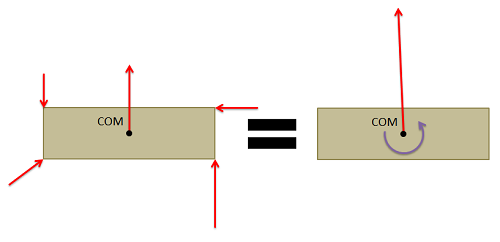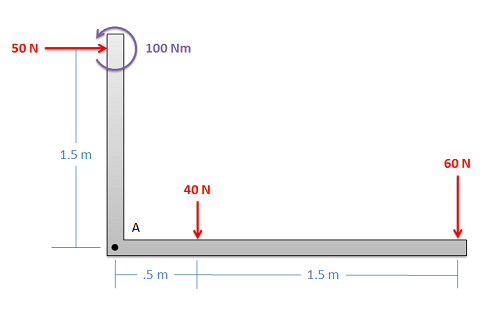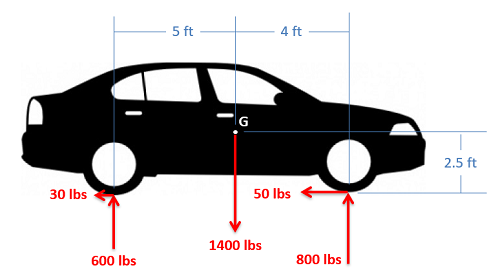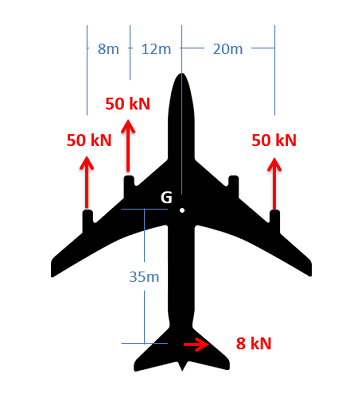﻿ Mechanics Map - Equivalent Force Couple System

# Equivalent Force Couple System

Every set of forces and moments has an equivalent force couple system. This is a single force and pure moment (couple) acting at a single point that is statically equivalent to the original set of forces and moments.Any set of forces on a body can be replaced by a single force and a single couple acting that is statically equivalent to the original set of forces and moments. This set of an equivalent force and a couple is known as the equivalent force couple system.

To find the equivalent force couple system, you simply need to follow the steps below.

1. First, choose a point to take the equivalent force couple system about. Any point will work, but the point you choose will affect the final values you find for the equivalent force couple system. Traditionally this point will either be the center of mass of the body or some connection point for the body.
2. Next resolve all the forces not acting though that point to a force and a couple acting at the point you chose.
3. To find the "force" part of the equivalent force couple system add together all the force vectors. This will give you the magnitude and the direction of the force in the equivalent force couple system.
4. To find the "couple" part of the equivalent force couple system, add together any moment vectors (this could be moments originally acting on the body or moments from the resolution of the forces into forces and couples). This will give you the magnitude and direction of the pure moment (couple) in the equivalent force couple system.

## Worked Problems:

### Question 1:

Find the equivalent force couple system about point A for the set of forces shown below.### Question 2:

Find the equivalent force couple system about point G for the set of forces shown below.### Question 3:

An engine on an airplane fails mid-flight. The pilot tries to compensate using the rudder at the back of the tail. Find the equivalent force couple system about point G for the set of forces shown on the plane.